# B.TECH 3rd sem Logic Design paper 2016

UniversityVTU, Belgaum, Karnataka
CourseB.TECH
Semester3
SubjectLogic Design
Year2016
Downloads1
Uploaded By###### Heman Sharma
100

 Question Marks 0 requests a. Explain the following canonical form:i) F(x, y, z) =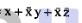This question has 0 answers so far. 5 0 requests Explain the following canonical form:ii) F(x, y, z) = (x + 2)(x+y)(y +z) This question has 0 answers so far. 5 0 requests b. Find the minimal POS expression of incompletely specified Boolean function using K-map ,f(a, b, c, d) -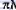M(1, 2, 3, 4, 9, 10) +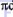d(0, 14, 15).  This question has 0 answers so far. 5 0 requests C. Find all the minimal SOP expression of f(a, b, c, d) -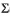(6, 7, 9, 10, 13) +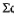d(1, 4, 5, 11, 1) using k-map.  This question has 0 answers so far. 5 0 requests a. Find all the prime implicants of the function:f(a, b, c, d) =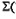(7, 9, 12, 13, 14, 15+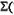d(4, 11) using Quine - MaClusky's algorithm.  This question has 0 answers so far. 10 0 requests (LU Marksb. For a given incomplete Boolearn function, find a minimal sum and minimal product expression using MEV technique taking least significant bit as map entered variable. f(a, b,c,d) =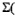(1, 5, 6, 7,971, 12, 13) +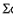d(0, 3, 4).  This question has 0 answers so far. 10 0 requests a. Implement the function using active low output dual 2 : 4 line decoder IC74139i) f1(A, B, C) =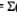m(0, 1, 2, 5) ii) f2(A, B,C) =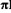M(1, 3, 4, 7).  This question has 0 answers so far. 10 0 requests b. Design priority encoder with three inputs, with middle bit at highest priority encoding to 10,most significant bit at next priority encoding to 11 and least significant at least priority ending 01.  This question has 0 answers so far. 10 0 requests a. Define multiplexer and demulitplexer and draw block diagram. This question has 0 answers so far. 4 0 requests b. Design 4 : 1 multiplexer, draw the circuit using gates. This question has 0 answers so far. 6 0 requests c. Explain how will you implement the following function using implementation table,F(A, B, C, D) =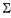m(0, 1, 3, 4, 7, 10, 12, 14) with A, B, C as select lines.  This question has 0 answers so far. 10 0 requests a. Design full adder and draw the circuit using two input NAND gates. This question has 0 answers so far. 7 0 requests b.Design and draw the circuit of look ahead carry generator using gates. Draw the block diagram of 4-bit parallel adder using look ahead carry generator.  This question has 0 answers so far. 10 0 requests c. Design single bit magnitude comparator and draw the circuit. This question has 0 answers so far. 3 0 requests a. Obtain the following for SR flip-flop:i) Characteristic equation ii) Excitation tableiii) State diagram. This question has 0 answers so far. 6 0 requests b. With the help of a schematic diagram, explain how a serial shift register can be transformed into a i) ring counter ii) Johnson counter. This question has 0 answers so far. 4 0 requests c. Design mod6 synchronous counter using D-flip-flops. This question has 0 answers so far. 10 0 requests a. A sequential network has one input and one output the state diagram is shown in Fig. 7(a).Design the sequential circuit using T flip-flops.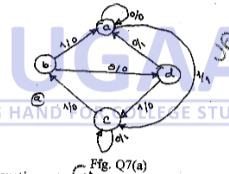This question has 0 answers so far. 10 0 requests (a)Derive the transition equations, transition table, state table and state diagram for the following: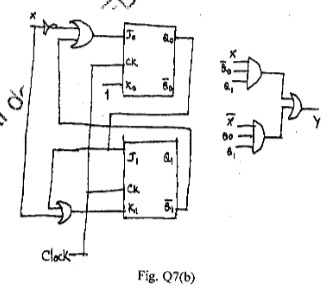This question has 0 answers so far. 10 0 requests Write notes on:a. Mealy and Moore model  This question has 0 answers so far. 10 0 requests Write notes on:b. State machine notation.  This question has 0 answers so far. 10
Chat with us